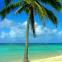Java Games: Flashcards, matching, concentration, and word search.# Triangle Terms

Match the term with the correct definition.

AB
right angleA 90 degree angle
equilateral triangleA triangle with all sides and angles equal.
scalene triangleA triangle having three unequal sides and angles.
vertexThe intersection point of two sides.
right triangleA triangle with one angle equal to 90 degrees.
acute angleAn angle less than 90 degrees.
obtuse angleAn angle greater than 90 degrees.
isosceles triangleA triangle with two sides of equal length.
acute triangleA triangle with all angles less than 90 degrees.
obtuse triangleA triangle with one angle greater than 90 degrees.Mrs. Budny Resource Teacher Montrose Elementary Henrico, VA

 This activity was created by a Quia Web subscriber. Learn more about QuiaCreate your own activities